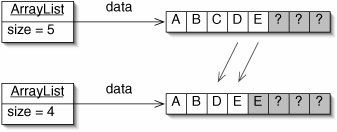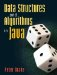# The List Interface

The Stack and Queue interfaces, while useful, are somewhat limited. We can only get at the ends of such data structures. We cannot, for example, easily search through a Stack for some element. In this section, we present and implement the List interface, which describes a more general-purpose linear sequence.

The Interface

A List is a sequence of elements. The first element is element 0, the next is element 1, and so on. It is similar to an array, but it does not have a fixed size. Several methods are required of any List implementation. The List interface is given in Figure 5-25.

The behavior of most of the methods is explained in the comments.

We will implement the List interface with a class ArrayList. The constructor for ArrayList produces an initially empty List. Thus, if we declare

```List list = new ArrayList();
```

Figure 5-25. The List interface. The Iterable interface is discussed in Section 5.4.

 ``` 1 /** A list of elements. */ 2 public interface List extends Iterable { 3 4 /** Add target to the back of this List. */ 5 public void add(E target); 6 7 /** Return true if some item in this List equals() target. */ 8 public boolean contains(E target); 9 10 /** Return the indexth element of this List. */ 11 public E get(int index); 12 13 /** Return true if this List is empty. */ 14 public boolean isEmpty(); 15 16 /** 17 * Remove and return the indexth element from this List, shifting 18 * all later items one place left. 19 */ 20 public E remove(int index); 21 22 /** 23 * Remove the first occurrence of target from this List, shifting 24 * all later items one place left. Return true if this List 25 * contained the specified element. 26 */ 27 public boolean remove(E target); 28 29 /** Replace the indexth element of this List with target. */ 30 public void set(int index, E target); 31 32 /** Return the number of element in this List. */ 33 public int size(); 34 35 } ```

and then

```list.add("eggs");
```

then list is the ArrayList that is printed as [ eggs bread tea ].

At this point, list.contains("eggs") is true, but list.contains("rutabagas") is false.

The expression list.get(1) returns "bread" because, as with arrays, list indices start at 0.

Not surprisingly, list.isEmpty() returns false.

The expression list.remove(0) both modifies list to be [ bread tea ] and returns "eggs".

If we perform the invocation list.remove("llamachow") on the resulting List, no change is made and false is returned. On the other hand, list.remove("tea") reduces list to [ bread ] and returns true.

Since there is only one element left, list.size() returns 1.

The ArrayList Class

We now implement the List interface with the ArrayList class. Like an ArrayStack, an ArrayList has two fields, data and size (Figure 5-26).

Figure 5-26. Fields and constructor for the ArrayList class.

 ``` 1 /** An array-based List. */ 2 public class ArrayList implements List { 3 4 /** Array of elements in this List. */ 5 private E[] data; 6 7 /** Number of elements currently in this List. */ 8 private int size; 9 10 /** The List is initially empty. */ 11 public ArrayList() { 12 data = (E[])(new Object); // This causes a compiler warning 13 size = 0; 14 } 15 16 } ```

Several of the ArrayList methods (Figure 5-27) are either trivial or identical to methods from ArrayStack.

The first interesting method is contains() (Figure 5-28). To determine whether an ArrayList contains some item, we work our way down data, comparing each element to the target. If we find it, we return TRue. If we get to the end of the "in use" portion of data, we return false.

The toString() method uses a similar for loop (Figure 5-29).

As specified by the List interface, there are two overloaded remove() methods (Figure 5-30). The first one removes and returns the item at a particular location. The second one removes the first occurrence of a particular item, if any.

Figure 5-27. These methods from ArrayList are either trivial or identical to methods from ArrayStack.

 ``` 1 public void add(E target) { 2 if (isFull()) { 3 stretch(); 4 } 5 data[size] = target; 6 size++; 7 } 8 9 public boolean isEmpty() { 10 return size == 0; 11 } 12 13 /** Return true if data is full. */ 14 protected boolean isFull() { 15 return size == data.length; 16 } 17 18 public E get(int index) { 19 return data[index]; 20 } 21 22 public void set(int index, E target) { 23 data[index] = target; 24 } 25 26 public int size() { 27 return size; 28 } 29 30 /** Double the length of data. */ 31 protected void stretch() { 32 E[] newData = (E[])(new Object[data.length * 2]); // Warning 33 for (int i = 0; i < data.length; i++) { 34 newData[i] = data[i]; 35 } 36 data = newData; 37 } ```

In both cases, all subsequent elements are shifted one place to the left. This shifting is illustrated in Figure 5-31.

The remaining method, iterator(), is explained in Section 5.4.

Figure 5-28. In contains(), each element is compared with target.

 ``` 1 public boolean contains(E target) { 2 for (int i = 0; i < size; i++) { 3 if (data[i].equals(target)) { 4 return true; 5 } 6 } 7 return false; 8 } ```

Figure 5-29. The toString() method.

 ```1 public String toString() { 2 String result = "[ "; 3 for (int i = 0; i < size; i++) { 4 result += data[i] + " "; 5 } 6 return result + "]"; 7 } ```

Figure 5-30. ArrayList has two overloaded remove() methods. On a successful removal, both require that later Objects be shifted left.

 ``` 1 public E remove(int index) { 2 E result = data[index]; 3 for (int i = index + 1; i < size; i++) { 4 data[i - 1] = data[i]; 5 } 6 size--; 7 return result; 8 } 9 10 public boolean remove(E target) { 11 for (int i = 0; i < size; i++) { 12 if (data[i].equals(target)) { 13 for (int j = i; j < size - 1; j++) { 14 data[j] = data[j + 1]; 15 } 16 size--; 17 return true; 18 } 19 } 20 return false; 21 } ```

Figure 5-31. In the ArrayList above, the element C (at position 2) is to be removed. This requires each subsequent element to be shifted left one position.Exercises

 5.15 Explain what's wrong with the version of contains() in Figure 5-32.

Figure 5-32. Broken version of contains() for Exercise 5.15.

 ``` 1 public boolean contains(E target) { 2 for (E e : data) { 3 if (e.equals(target)) { 4 return true; 5 } 6 } 7 return false; 8 } ```
 5.16 The List interface specifies two overloaded remove() methods. Through experimentation, determine which one Java uses if we invoke remove(3) on a List. How can we force Java to use the other one? 5.17 Modify the get() and set() methods (Figure 5-27) so that they throw an IndexOutOfBoundsException if the argument index is either too low (less than 0) or too high (greater than or equal to size). (IndexOutOfBoundsException is the direct superclass of ArrayIndexOutOfBoundsException.) 5.18 Explain why the loop in the first version of remove() (Figure 5-30) starts at index + 1 instead of index. 5.19 Write an equals() method for the ArrayList class. This method should compare only elements in the "in use" region of data. 5.20 Write a method indexOf() that takes one argument, an element target, and returns the index of the first occurrence of target in this ArrayList, or -1 if target does not appear. 5.21 Write a method removeAll() that takes one argument, an element target. It removes all elements which are equals() to target from this ArrayList. 5.22 Write a method count() that takes one argument, an element target. It returns the number of elements in this ArrayList which are equals() to target. 5.23 Write a main() method to test the ArrayList class.Data Structures and Algorithms in Java
ISBN: 0131469142
EAN: 2147483647
Year: 2004
Pages: 216
Authors: Peter Drake